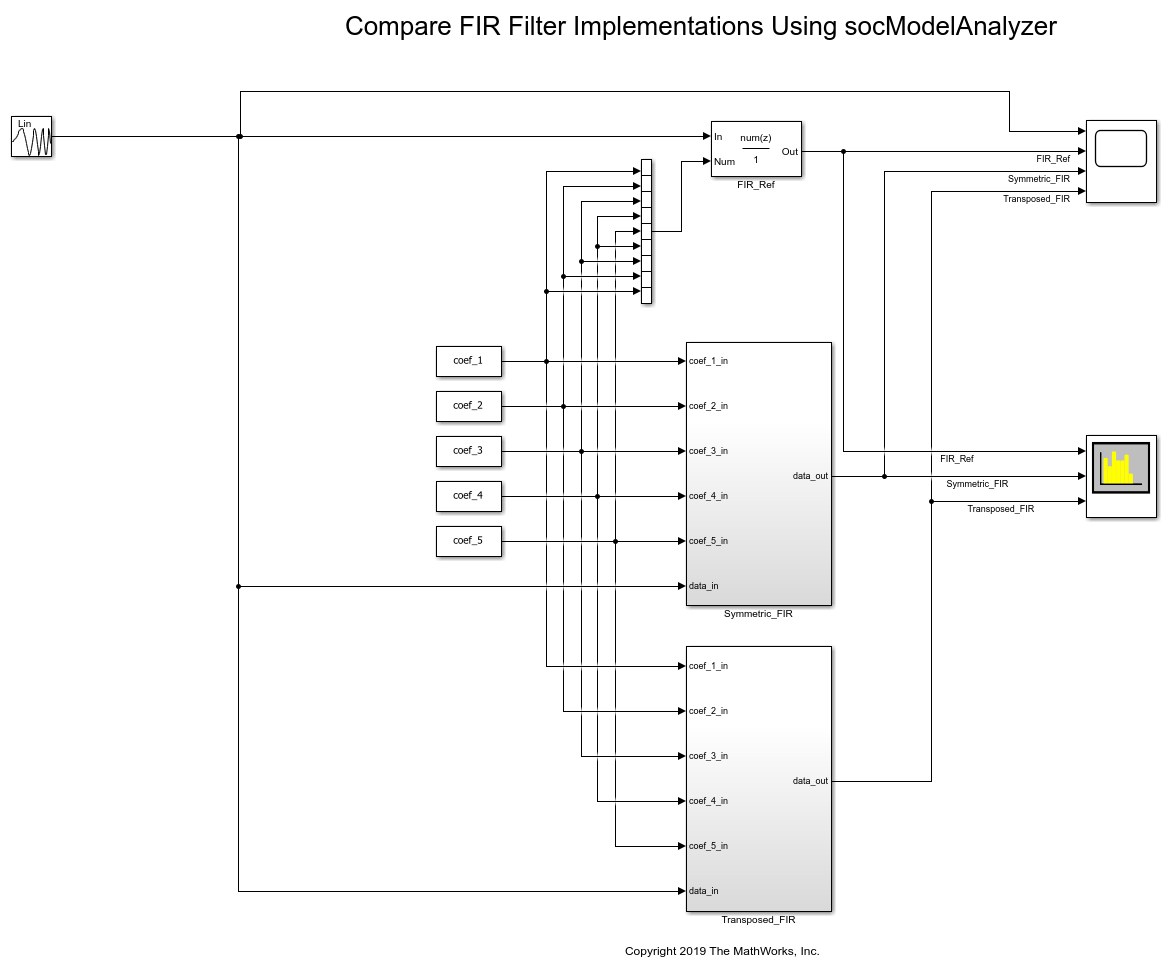# Compare FIR Filter Implementations Using socModelAnalyzer

This example shows how to analyze and compare different implementations of a Simulink® algorithm based on the number of arithmetic operations. Use the SoC Blockset `socModelAnalyzer` function to generate reports that show the number of operators for different implementations of a FIR Filter using static and runtime execution.

This design task evaluates two implementations of a FIR filter and compares the implementation costs. This example uses the number of operators as a way to measure the implementation cost.

To meet system requirements such as speed, latency, and hardware resources, consider and compare several implementations of the algorithm. The number of arithmetic operators used in an implementation can help you identify resource usage and allocation.

Manual analysis and calculation of the number of arithmetic operators can be tedious, error prone, and time consuming. Manual calculations can be inaccurate for an algorithm involving a branch, loop, or recursion construct and might be impossible to calculate if the execution path depends on the input data or random factors (for example, a convergence algorithm).

### Structure of Model

The `soc_analyze_FIR_tb` model implements a low pass digital FIR filter in two ways. The Symmetric_FIR subsystem exploits symmetry in coefficients to optimize the resources. The Transposed_FIR subsystem employs a filter structure geared toward higher speed of operation. The model uses a chirp input signal as an input stimulus and a FIR_ref (Discrete FIR Filter) block as a reference for checking numerical correctness of the implementations.

Open the `soc_analyze_FIR_tb` model in Simulink and examine the structure of the model.

```open_system('soc_analyze_FIR_tb'); ```To design the low pass FIR filter we use the `filterDesigner` (DSP System Toolbox) app to generate coefficients for an 8th order FIR filter. The FIR filter has a cutoff frequency of 0.25 (normalized) and a passband ripple and stop band attenuation of 1 dB and 60 dB, respectively. The model sets these coefficients via the model initialize callback.

Simulate the model to validate the functionality of both implementations against the reference FIR block. The responses of the filter implementations match the reference.

```sim('soc_analyze_FIR_tb'); ```### Compare Implementations Using Model Analyzer

Use the `socModelAnalyzer` function to generate reports for the number of arithmetic operators in each implementation and compare the implementations. The reports are generated using the runtime execution of the model.

Symmetric FIR Filter:

To estimate the number of operators for Symmetric FIR filter implementation, use the `socModelAnalyzer` function. Specify the subsystem name for the `IncludeBlockPath` name-value pair argument of the function. Set the output folder to specify where to generate the reports. Enter this command at the MATLAB command prompt.

```socModelAnalyzer('soc_analyze_FIR_tb.slx','Folder','report_sym','IncludeBlockPath',... 'soc_analyze_FIR_tb/Symmetric_FIR'); ```
```Generating operators analysis report for /tmp/Bdoc21b_1757077_76220/tp708e84ab/ex82446029/soc_analyze_FIR_tb.slx ... Saving report files in /tmp/Bdoc21b_1757077_76220/tp708e84ab/ex82446029/report_sym. Operator estimate: <a href="matlab: socAlgorithmAnalyzerReport('/tmp/Bdoc21b_1757077_76220/tp708e84ab/ex82446029/report_sym/soc_analyze_FIR_tb.mat')">Open report viewer</a> Done. ```Open the report by clicking the Open report viewer link on the MATLAB console. Alternatively, you can use the `socAlgorithmAnalyzerReport` function. The report provides two views. The first view is the operator view, which presents the data such that each row corresponds to an operator. To use this view, click Operator View on the report toolstrip. The second view is the model view where each row corresponds to a Simulink subsystem path. To use this view, click Model View on the report toolstrip. Reports are also saved in the `report_sym` folder as a MAT-file (`soc_analyze_FIR_tb.mat`) and an Excel® file (`soc_analyze_FIR_tb.xlsx`).

By default, the report opens with the operator view. The viewer opens the aggregate view of each operator and data type. For example, the Symmetric FIR filter contains a total of 8 `ADD(+)` operators of data-type `double` and 5 `MUL(*)` operators of data-type `double` executed 10,001 times each. (The model simulation duration is 10 s and the base rate is 10 ms. This produces 10,000 simulation cycles plus 1 for initialization.) To get the detailed report for each operator, expand that operator. The report shows the operator count as used in various blocks. Trace the operator by clicking on one of the links in the last column of the report to highlight the location of the operator in the `soc_analyze_FIR_tb` model.Transposed FIR Filter

To estimate the number of operators for the Transposed FIR filter implementation, use the `socModelAnalyzer` function. Specify `'soc_analyze_FIR_tb/Transposed_FIR'` for the `'IncludeBlockPath'` name-value pair argument of the function. Set the output folder for the generated reports to `report_trans`. Enter this command at the MATLAB command prompt.

```socModelAnalyzer('soc_analyze_FIR_tb.slx','Folder','report_trans','IncludeBlockPath',... 'soc_analyze_FIR_tb/Transposed_FIR'); ```
```Generating operators analysis report for /tmp/Bdoc21b_1757077_76220/tp708e84ab/ex82446029/soc_analyze_FIR_tb.slx ... Saving report files in /tmp/Bdoc21b_1757077_76220/tp708e84ab/ex82446029/report_trans. Operator estimate: <a href="matlab: socAlgorithmAnalyzerReport('/tmp/Bdoc21b_1757077_76220/tp708e84ab/ex82446029/report_trans/soc_analyze_FIR_tb.mat')">Open report viewer</a> Done. ```Open the report for the Transposed FIR filter by clicking the Open report viewer link on the MATLAB console.For the Transposed FIR filter, the report shows an estimated number of 8 additions of data-type `double` and 9 multiplications of data-type `double` (each operator executed 10,001 times).

Comparison of Symmetric and Transposed Implementations

Compare the symmetric and transposed FIR filter reports generated by using the `socModelAnalyzer` function. The Symmetric FIR filter uses fewer multiplication operators (9) than the Transposed FIR filter (5). They both use the same number of add operators (8).

### Conclusion

You used the `socModelAnalyzer` function to estimate and analyze the number of arithmetic operators in two FIR filter implementations. You generated operator reports for both Symmetric and Transposed FIR filters. You compared the number of multiply and add operators for two implementations.

You can use the `socModelAnalyzer` function for analyzing the number of operators in a Simulink algorithm.